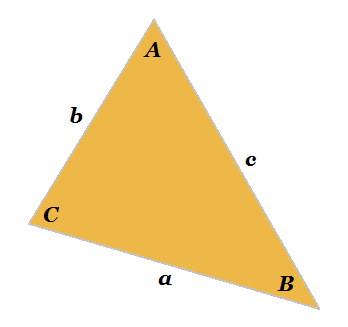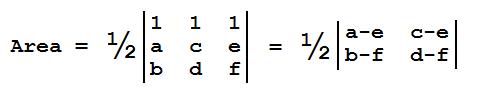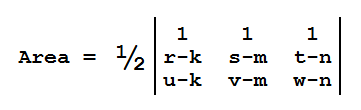# 3-Coordinate Triangle Calculator

Coordinate Triangle Calculator
2-d      3-d

Points: ( , )
A = ( , )
B = ( , )
C = ( , )

In 2-, 3-, or n-dimensional coordinate space, a triangle is defined by three non-collinear points. If you know the coordinates of these points, you know everything there is to know about the triangle, including its area, angles, and side lengths. The easiest aspect to compute from the coordinates is the area, since it can be calculated from a matrix determinant.

You can use the calculator on the left to compute triangular metrics in 2- or 3-dimensional space. The matrix method for computing area is described below.### 2-Dimensional Coordinates (x, y)

The area of a triangle given by a set of three 2-dimensional coordinates (a, b), (c, d), (e, f) is the matrix determinant:This is because the area of a parallelogram formed by the vectors <a-e, b-f> and <c-e, d-f> is equal to the determinant of a matrix with the rows (or columns) [a-e, b-f] and [c-e, d-f]. The area of the triangle is simply half the area of the parallelogram.

### 3-Dimensional Coordinates (x, y, z)

Suppose a triangle in 3-space has coordinates (k, m, n), (r, s, t), and (u, v, w). Let P be the vector <r-k, s-m, t-n> and let Q be the vector <u-k, v-m, w-n>. The absolute value of the cross product of P and Q is given by the equation

|P × Q| = sin(θ)|P||Q|,

where θ is the angle between P and Q. The right hand side is twice the formula for the area of a triangle. Thus, the area of the triangle formed by the three points is (1/2)|P × Q|. This can be represented by a matrix determinant as well: